台北 路境行旅(原謙商旅-總統府館) CHAIIN HOTEL – TZUNG TUNG FU

2,475

• 捷運台大醫院站
• 新光三越百貨 站前店

選擇專案房型及間數

更改 台北 路境行旅(原謙商旅-總統府館) 的空房情形及價格

•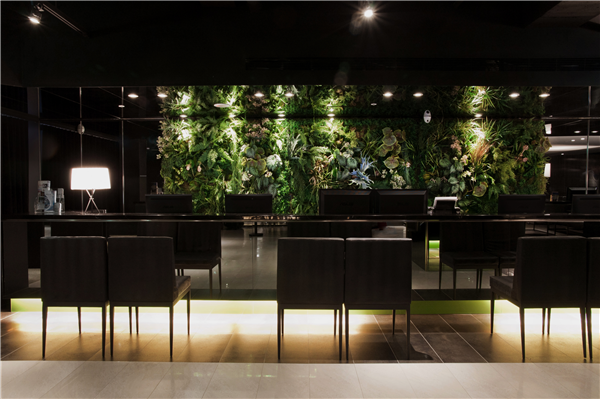接待處
•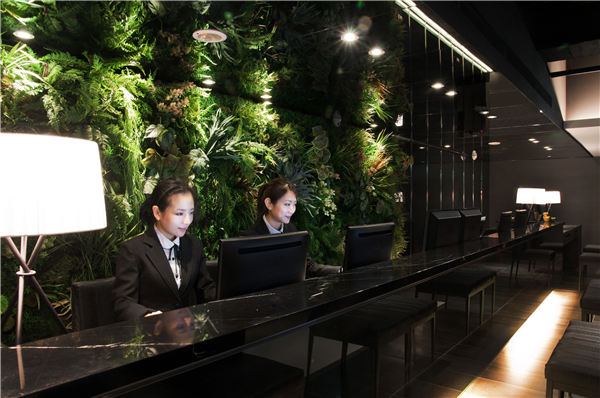接待處
•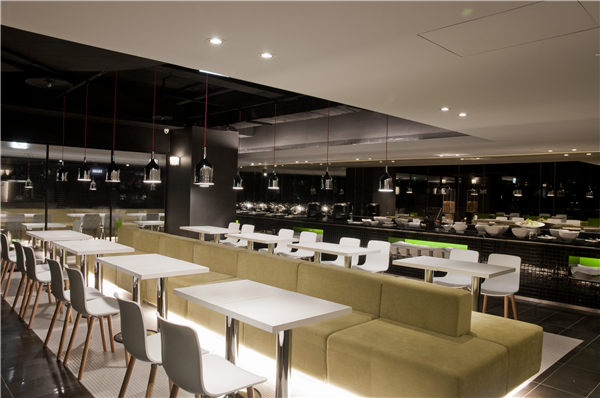餐廳
•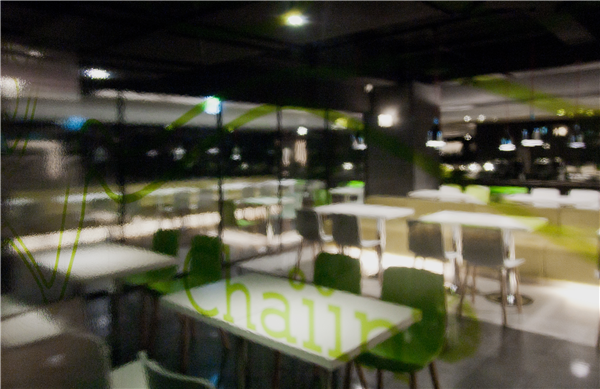餐廳
•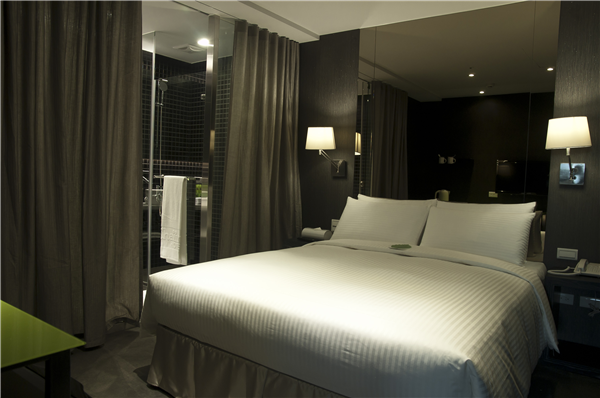客房
•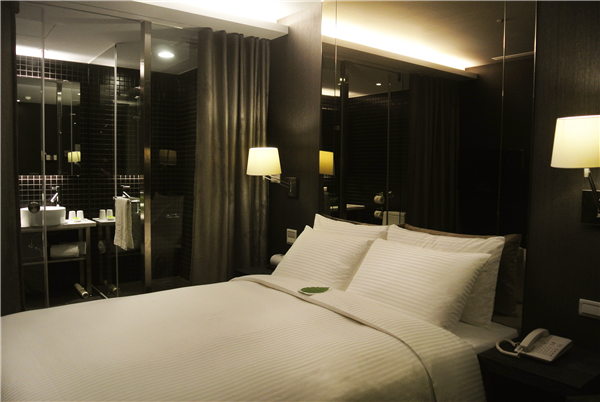客房
•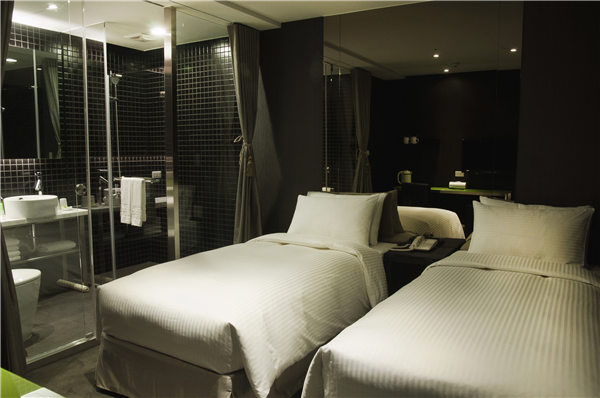客房
•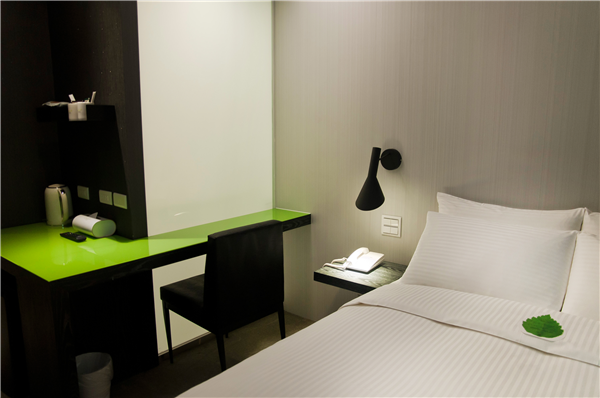客房
•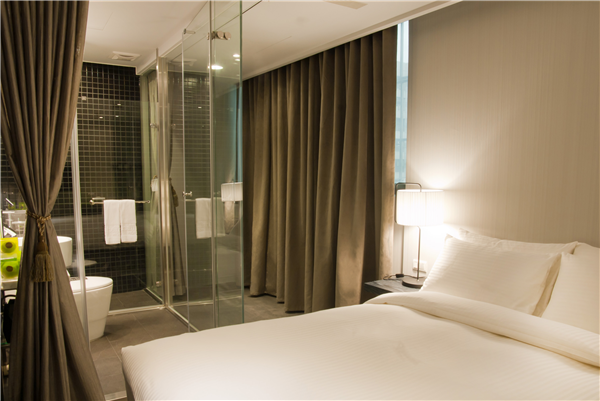客房
•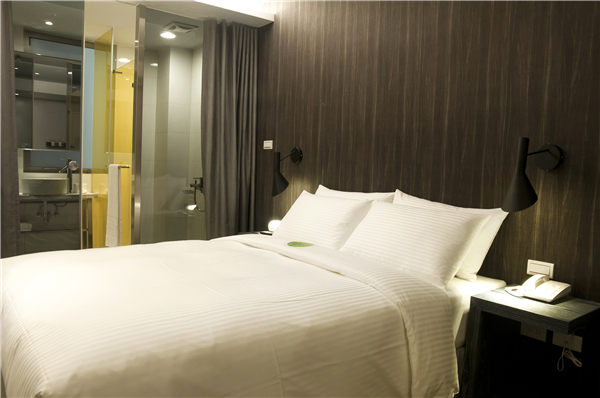客房
•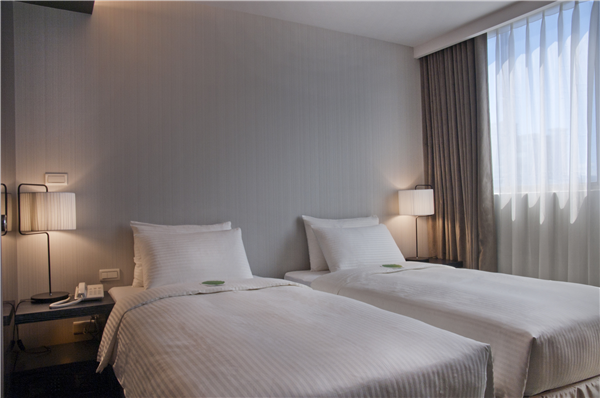客房
•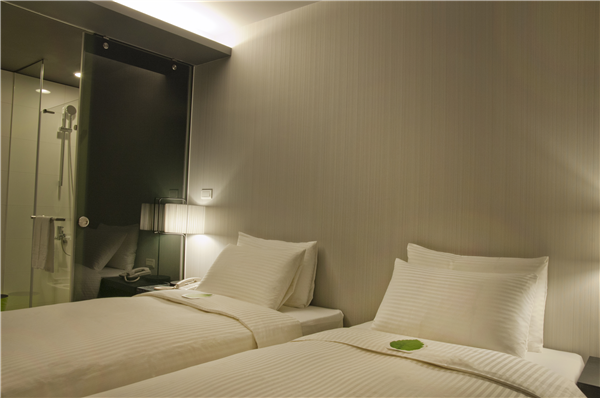客房
•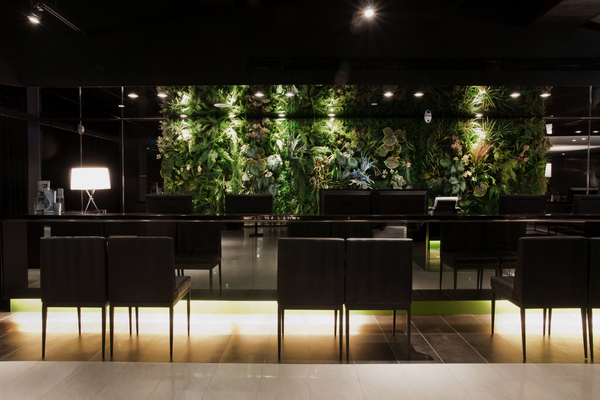環境
•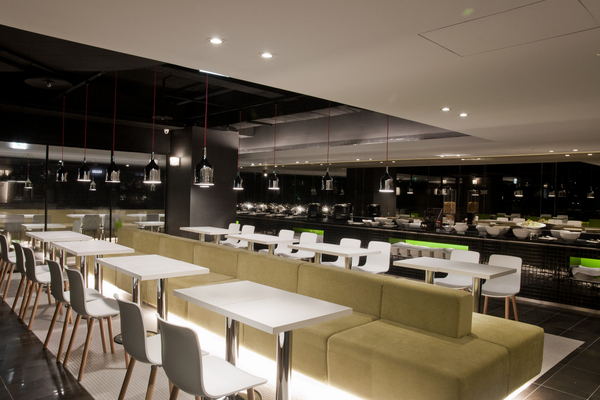環境
•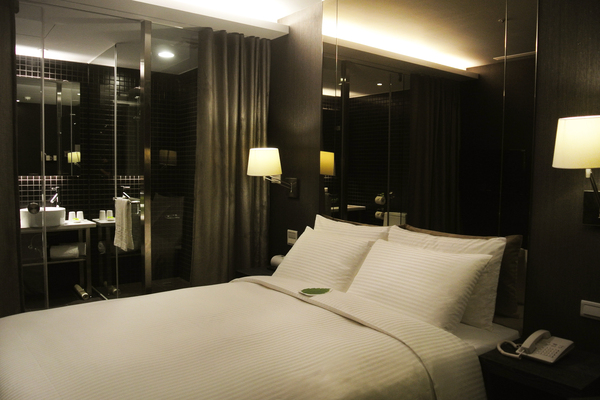客房
•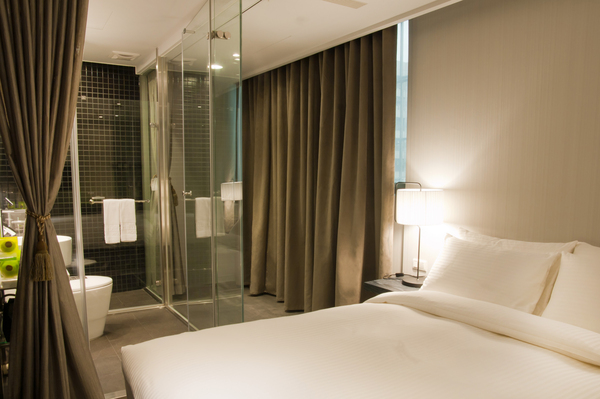客房

飯店設有　 飯店未設 飯店設施

• 24小時客房服務
• 商務中心
• 洗衣服務/乾洗
• Wi-Fi in public areas
• 夜總會
• 禮賓接待服務
• 代客泊車
• 娛樂場
• 自行車出租服務
• 保管服務
• 客房服務
• 行政樓層
• 停車場
• 家庭房
• 酒吧
• 兒童看護服務
• 接送服務
• 電梯
• 可帶寵物
• 旅遊服務
• 飯店/機場接送
• 吸煙房
• 會議設施
• 餐廳
• 咖啡店
• 殘障人士設施
• 髮廊
• 商店
• 池畔吧

• DVD/CD播放器
• 小廚房
• 無線上網(收費)
• 休憩區
• 微波爐
• 燙衣板
• 免費瓶裝水
• 房內保險箱
• 獨立淋浴間
• 公共浴室
• 房內遊戲機
• 獨立用餐區
• 冰箱
• 手提電腦保險箱
• 禁煙房
• 冷氣
• 按摩浴缸
• 私人泳池
• 加長的床(>2m)
• 日報
• 茶與咖啡沖泡設備
• 可提供相連房間
• 書桌
• 衛星頻道/有線電視
• 吹風機
• 洗衣機
• 迷你吧
• 寬頻上網
• 浴室
• 陽台/露台
• 寬頻上網(免費)
• 浴缸
• 電影點播服務
• 寬頻上網(收費)
• 浴袍
• 電視
• 寬頻上網(無線)
• 液晶電視
• 電風扇
• 尊用休息室
• 無線上網(免費)

• spa
• 三溫暖/水療設備
• 網球場
• 健身房/健身設施
• 水上活動
• 花園
• 兒童娛樂室
• 水上運動項目(非機動項目)
• 蒸浴室
• 壁球場
• 溫泉
• 遊戲室/娛樂場
• 室內游泳池
• 游泳池(兒童)
• 高爾夫球場(3km內)
• 室外游泳池
• 漩渦按摩浴池
• 高爾夫球場(飯店)
• 按摩
• 私人海灘

飯店條款

• 加床政策根據您所選定的客房而有所不同，更多詳情請查看您所選定的客房的政策

相關資訊

樓層總數 6 3:00 PM 2013 2013 110V 158間

0
0